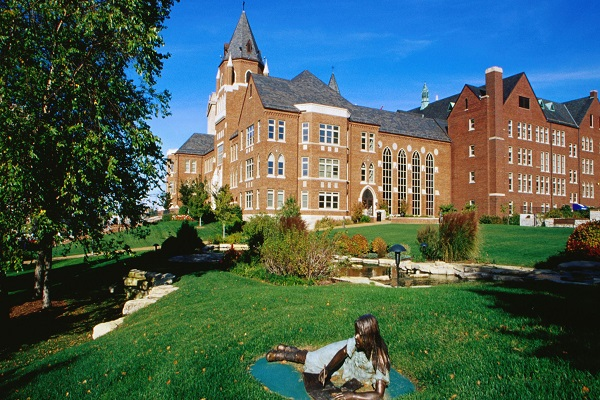## BS Mathematics

#### University of Missouri-St. Louis

##### St. Louis, Missouri, USADuration 4 Years Bachelors Program
• The B.S. in Mathematics program provides a substantial background in mathematics, statistics, and computer science to produce graduates who can work as mathematicians.

• The program allows optional courses that enable the student to focus on areas of interest like pure or applied mathematics.

• The following course work in the Department of Mathematics and Statistics is required:
• Introduction to Computing
• Introduction to Probability and Statistics
• Analytic Geometry and Calculus I
• Analytic Geometry and Calculus II
• Analytic Geometry and Calculus III
• Introduction to Differential Equations
• Elementary Linear Algebra
• Foundations of Mathematics
• Design and Analysis of Algorithms
• Real Analysis I
Fees componentsAmount
Tuition & fees2414503 INR
Hostel & Meals762502 INR
Insurance153877 INR
Transportation36620 INR
Books & Supplies73240 INR
Personal Expenses310391 INR